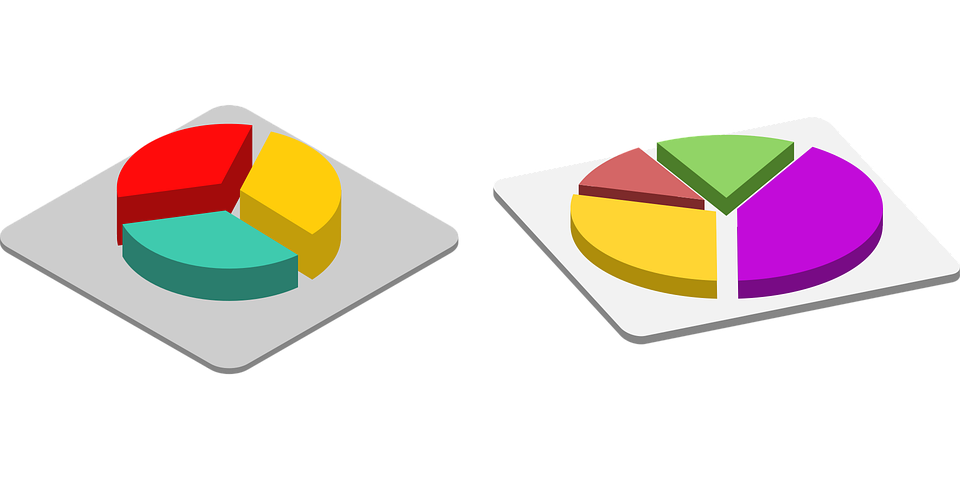# Pie Chart

Pie charts are different types of data presentation. This data is presented in the form of a circle. There are segments and sectors into which a pie chart is being divided and each of these segments and sectors forms a certain portion of the total(in terms of percentage). In the pie-chart, the total of all the data is equal to 360 degrees. The degree of angles that are used to represent different items are calculated in the form of proportionality. Let us know more about them.

## Pie charts

In pie charts, the entire diagram looks like a pie and the components in it resembles the various slices cut from the pie. The pie-chart is thus used to show the break-up of one continuous variable into its components parts.Source: Pixabay

For, the example you can see the distribution of sales of the laptop industry between five companies:Company % in market share HP 22 Dell 33 Lenovo 13 Asus 15 Acer 17

The pie chart represented above shows a circle of 360 degrees which represents 100 % of the values of the continuous variable. Thus, 3.6 degree of the pie chart represents 1 % of the total values of the variables being represented. Pie-charts are more versatile than the bar graphs, tables or two variables graphs. It also represents the clear and precise data that needs to display. Thus, also helps you establish the relationship between different sectors.

### Solved Examples

Directions:

The following pie-chart shows the market share of different banks in India. Based on this pie-chart determine the questions given below.

1. If the value of the market share of BOI is Rs. 4000 crores, then the market share of BOB and Axis bank together is:A. 24,363 crores
B. 24,432 crores
C. 24,864 crores
D. 25, 827 crores

This is the most common question asked in the pie chart section. You will be given the share price and based on its comparison with the percentage given in the question you need to determine the answer. Thus, in this question, you can see that BOI accounts for 11 % of the market share. And this 11 % is equal to the 4000 crores. So to calculate the total market share of BOB and axis the formula will be, 67/11 x 4000 = 24,363. Thus, the correct answer is A.

2. If the total market share other than Axis and BOB is Rs. 335,000 crores. Then find the market share of BOI and HDFC banks.

A. 274,560 crores
B. 274,090 crores
C. 274,809 crores
D. Cannot be determined

Here, from the pie-chart, you can determine that the total market share of Axis and BOB in terms of percentage is 67 % and this is equal to Rs. 335,000. In addition to this the other banks that are left accounts for 33 % which equates to 35,000 crores. HDFC and BOI equal the market share of 27 %. Thus, their market share in terms of crores is 27 x 335000/33 => 274090 crores. So, the correct answer is C.

### More Questions

3. Find the approximate ratio of market share between BOI and BOB.

A. 1: 2
B. 3: 1
C. 1 : 3
D. Not possible

Take the market share of BOB and BOI and compare them both to find the ratio. As a result, the market share of BOB is given as 34 % and the market share of BOI is given as 11 %. So, the ratio of market share of BOI to a market share of BOB will be 11: 34. This will be close to 1 : 3. So, the correct answer is C.

4. If the market share of Axis banks doubles in the next year while the market share of all the other banks remains constant at their existing levels, then what would be the proportion of Axis as the total proportion of the market share in India in next year?

A. 45 %
B. 50 %
C. 55 %
D. 62 %

We need to determine the market share Axis bank for next year. For the current year, the share of Axis bank is 33 %. So now we need to determine the next year share of Axis bank. It is given that this share of Axis bank will be doubled while the shares of the other banks are constant. So the total percentage of all the shares will be 133 because Axis bank will 66 % of the market share. 66 is 50 % of 133, so the market share of Axis bank in the next year will be 50 %. Thus, the correct answer is B.

## Practice Questions

Direction:

The following pie-chart shows the distribution of the household items used in the house throughout the year. The information given is in terms of percentage. Study the graph and answer the questions below:Q. Which of the following items are displayed in the ratio of 1:15.

A. Food items and cosmetics
B. Technology and Food items
C. Cosmetics and others
D. Cosmetics and Food items

2. If the budget of the house is Rs. 20,000, then by what percentage is the expenditure on food items more than the rest of the expenditure?

A. Less than 10 %
B. 10 – 15 %
C. 15 – 20 %
D. More than 20 %

3. If the value of the next budget is twice that of the current one with the break-up of the items being the same, then the ratio of the expenditure on clothes to that of others will be near to…

A. 3: 4
B. 4: 5
C. 5: 6
D. 6: 7

4. If the budget value is Rs. 14,400, then the expenditure on clothes will be near to?

A. Rs. 1800
B. Rs. 2000
C. Rs. 2100
D. Rs. 2200